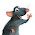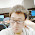### leetcode Question 97: Set Matrix Zeros

Set Matrix Zeros

Given a m x n matrix, if an element is 0, set its entire row and column to 0. Do it in place.
Did you use extra space?
A straight forward solution using O(mn) space is probably a bad idea.
A simple improvement uses O(m + n) space, but still not the best solution.
Could you devise a constant space solution?

Analysis:
To reduce the space required, we can use the matrix itself to store the flags for each row and column if they need to set to 0. So we need 1 row and 1 column, the 1st row and 1st column then can be chosen to store the flag. But we need first check the two if they need to be 0. Then go the other rows and columns.

e.g.

1 0 2 3 4 5
2 0 2 3 4 5
3 1 2 3 4 5

First check 102345 and
1
2
3
use two flags storing the status.  fr0 = true, fc0=false;
then check the rest of matrix, use the 1st col and 1st row store the status.
1 0 2 3 4 5
0 0 2 3 4 5

1 1 2 3 4 5
Then set 0s to sub-matrix(excludes 1st row and 1st column), according to the values in 1st row and 1st column, and finally set 1st row and 1st column according to flags.

The new space used is O(1+1) = O(1).

Code:
class Solution {
public:
void setZeroes(vector<vector<int> > &matrix) {
// Start typing your C/C++ solution below
// DO NOT write int main() function
int row = matrix.size();
if (row==0){return;}
int col = matrix.size();
if (col==0){return;}

bool fc0=false;
bool fr0=false;

for (int i=0;i<col;i++){
if (matrix[i]==0){fr0 = true;}
}

for (int i=0;i<row;i++){
if (matrix[i]==0){fc0 = true;}
}

for (int i=1;i<row;i++){
for (int j=1;j<col;j++){
if (matrix[i][j]==0){
matrix[i]=0;
matrix[j]=0;
}
}
}

for (int i=1;i<col;i++){
if (matrix[i]==0){
for(int j=0;j<row;j++){
matrix[j][i]=0;
}
}
}

for (int i=1;i<row;i++){
if (matrix[i]==0){
for(int j=0;j<col;j++){
matrix[i][j]=0;
}
}
}

if (fr0){
for (int i=0;i<col;i++){matrix[i]=0;}
}
if (fc0){
for (int i=0;i<row;i++){matrix[i]=0;}
}

return;

}
};


1.请问可否这样做：
第一次扫描全matrix，如果遇到0就把该行该列设成2，注意这时如果该列/该行有别的元素是0，那不设成2，这样扫描一遍后，就知道凡是2或0的位置就要设成0
第二遍再把它们都设成0

谢谢

1.what if there is several 2s in the matrix?
How do you know which 2s are from the original matrix or from your set?

2.This comment has been removed by the author.